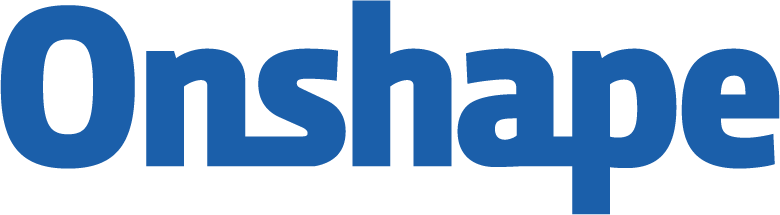Welcome to the Onshape forum! Ask questions and join in the discussions about everything Onshape.

First time visiting? Here are some places to start:
1. Looking for a certain topic? Check out the categories filter or use Search (upper right).
2. Need support? Ask a question to our Community Support category.
3. Please submit support tickets for bugs but you can request improvements in the Product Feedback category.
4. Be respectful, on topic and if you see a problem, Flag it.

If you would like to contact our Community Manager personally, feel free to send a private message or an email.

# grid of points inside a body by FS

I came to the forum looking for some help regarding a FS that I am trying to develop:
I would like to create a FS that creates a pseudo copy of a body but in a "lego-like form" (like a blockish version of it)
what I mean is to be able to recreate a body but with orthogonal blocs of the same size (a,b,c) one after each other.
for this, I thought about doing three loops for each ax (with a step of the size of the block in that direction)
and a conditional that if evaluateQuery(context, qContainsPoint(q, point)) != [] is true then create a bloc in that point (with fCuboid with vertex in point1(pointx-a/2,pointy-b/2,pointz-c/2) and vertex 2 in point2(pointx+a/2,pointy+b/2,pointz+c/2)
else do nothing.
I not sure how could I put the boundaries for the loops (they would be the smallest possible box that contains the body of sizes A,B,C) and the boundaries would be
for x [-(0.5*round(A/a)+1),0.5*round(A/a)+1]
for y [-(0.5*round(B/b)+1),0.5*round(B/b)+1]
for z [-(0.5*round(C/c)+1),0.5*round(C/c)+1]
Tagged:

• Member, Onshape Employees Posts: 8edited August 2019
Hey! Without too much optimization nor fiddling, I'd do something like the following feature. I'm computing the bounding box, the number of steps on each axis, and the corresponding point (offset by half a step).

annotation { "Feature Type Name" : "Rasterize" }
export const rasterize = defineFeature(function(context is Context, id is Id, definition is map)
precondition
{
annotation { "Name" : "Bodies", "Filter" : EntityType.BODY }
definition.bodies is Query;
annotation { "Name" : "Block size x" }
isLength(definition.blockSizeX, LENGTH_BOUNDS);
annotation { "Name" : "Block size y" }
isLength(definition.blockSizeY, LENGTH_BOUNDS);
annotation { "Name" : "Block size z" }
isLength(definition.blockSizeZ, LENGTH_BOUNDS);
}
{
// compute the bounding box
const bbox is Box3d = evBox3d(context, {
"topology" : definition.bodies,
"tight" : true
});

const minCorner = bbox.minCorner;
const maxCorner = bbox.maxCorner;
// [A, B, C]
const boxSize = maxCorner - minCorner;
// [a, b, c]
const blockSize = vector(definition.blockSizeX, definition.blockSizeY, definition.blockSizeZ);

for (var ix = 0; ix < boxSize / blockSize; ix += 1) {
const x = minCorner + (ix + 0.5) * blockSize;
for (var iy = 0; iy < boxSize / blockSize; iy += 1) {
const y = minCorner + (iy + 0.5) * blockSize;
for (var iz = 0; iz < boxSize / blockSize; iz += 1) {
const z = minCorner + (iz + 0.5) * blockSize;
const pt = vector(x, y, z);
if (size(evaluateQuery(context, qContainsPoint(definition.bodies, pt))) > 0)
{
// do something with pt and remove the debug
debug(context, vector(x, y, z));
}
}
}
}
});

• abovin said:
Hey! Without too much optimization nor fiddling, I'd do something like the following feature. I'm computing the bounding box, the number of steps on each axis, and the corresponding point (offset by half a step).

annotation { "Feature Type Name" : "Rasterize" }
export const rasterize = defineFeature(function(context is Context, id is Id, definition is map)
precondition
{
annotation { "Name" : "Bodies", "Filter" : EntityType.BODY }
definition.bodies is Query;
annotation { "Name" : "Block size x" }
isLength(definition.blockSizeX, LENGTH_BOUNDS);
annotation { "Name" : "Block size y" }
isLength(definition.blockSizeY, LENGTH_BOUNDS);
annotation { "Name" : "Block size z" }
isLength(definition.blockSizeZ, LENGTH_BOUNDS);
}
{
// compute the bounding box
const bbox is Box3d = evBox3d(context, {
"topology" : definition.bodies,
"tight" : true
});

const minCorner = bbox.minCorner;
const maxCorner = bbox.maxCorner;
// [A, B, C]
const boxSize = maxCorner - minCorner;
// [a, b, c]
const blockSize = vector(definition.blockSizeX, definition.blockSizeY, definition.blockSizeZ);

for (var ix = 0; ix < boxSize / blockSize; ix += 1) {
const x = minCorner + (ix + 0.5) * blockSize;
for (var iy = 0; iy < boxSize / blockSize; iy += 1) {
const y = minCorner + (iy + 0.5) * blockSize;
for (var iz = 0; iz < boxSize / blockSize; iz += 1) {
const z = minCorner + (iz + 0.5) * blockSize;
const pt = vector(x, y, z);
if (size(evaluateQuery(context, qContainsPoint(definition.bodies, pt))) > 0)
{
// do something with pt and remove the debug
debug(context, vector(x, y, z));
}
}
}
}
});

wow! thanks a lot for the help, i changed the "tight" to false to be faster. either way big thaaaanks a looot for the help.
I have another question, normally when I learned to program in a software, I always do the top down method, look what I wanted to do, similar examples and from there write my own code (or working with examples of each function, as in Matlab, for example...), but i couldnt find  really simple examples (is there anywhere?) and from the standard library I am pretty sure that I don't know how to find things in it like know what is capable and already coded or not... (good example is the evbox3D...) either way big thanks• Moderator, Onshape Employees, Developers Posts: 1,570edited September 2019
@Otaola_Franco

This is the homepage for all our FeatureScript resources:

There are some tutorials, and some articles about the top-level language features.

The documentation for every function in the language is here: